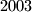### IMO Shortlist 2006 problem C2

Kvaliteta:
Avg: 0,0
Težina:
Avg: 6,0
Let$P$ be a regular$2006$-gon. A diagonal is called good if its endpoints divide the boundary of$P$ into two parts, each composed of an odd number of sides of$P$. The sides of$P$ are also called good.
Suppose$P$ has been dissected into triangles by$2003$ diagonals, no two of which have a common point in the interior of$P$. Find the maximum number of isosceles triangles having two good sides that could appear in such a configuration.
Izvor: Međunarodna matematička olimpijada, shortlist 2006